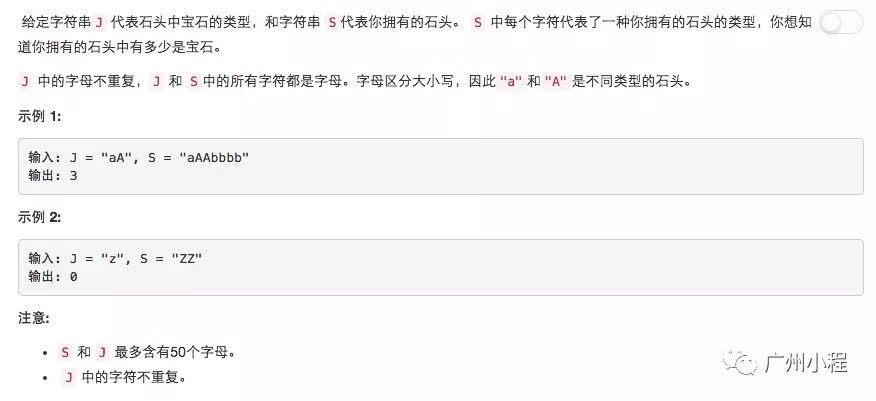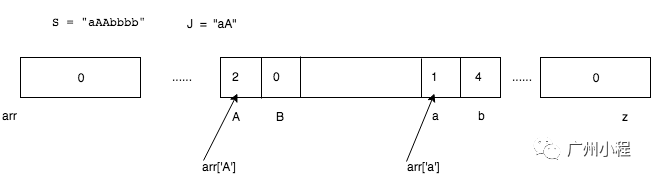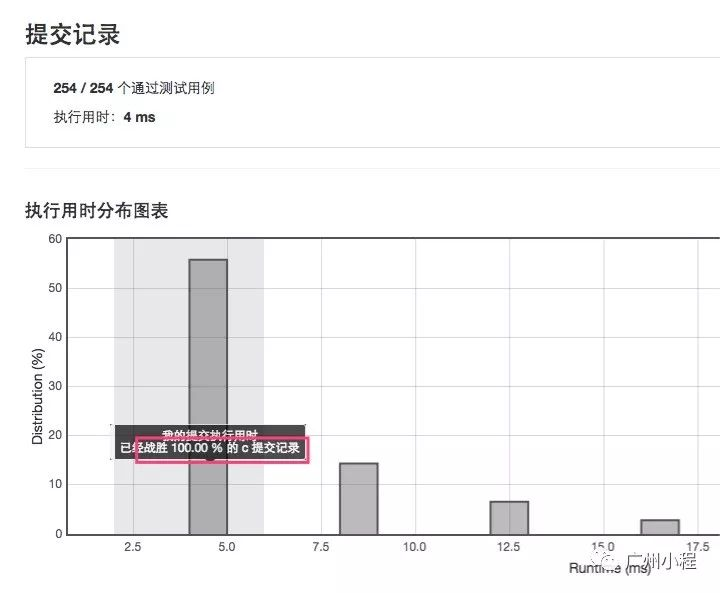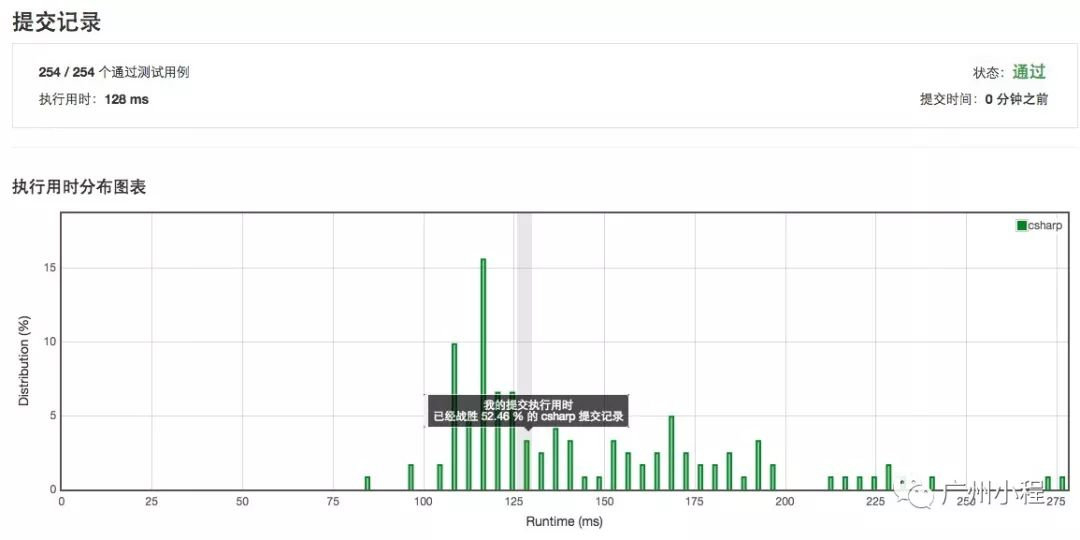# 算法演练1：钻石与石头

02/07 11:20

leecode是一个可以进行算法答题的网站，地址是：https://leetcode.com/，最近还出了中文版，地址是：https://leetcode-cn.com/// c code

#include <stdlib.h>

#include <string.h>

#include <stdio.h>

int numJewelsInStones(char* J, char* S) {

int arrlen = 'z'+1;

char* arr = (char*)malloc(arrlen);

memset(arr, 0, arrlen);

for (int i = 0; i < strlen(S); i ++) {

arr[S[i]] += 1;

}

int cnt = 0;

for (int j = 0; j < strlen(J); j ++) {

cnt += arr[J[j]];

}

free(arr);

return cnt;

}

int main(int argc, char *argv[])

{

char *S = "aAAbbbb", *J = "aA";

int cnt = numJewelsInStones(J, S);

printf("cnt=%d\n", cnt);

return 0;

}// c# code

public int NumJewelsInStones(string J, string S)

{

int result = 0;

if (string.IsNullOrEmpty(J) || string.IsNullOrEmpty(S)) return result;

var kv = new Dictionary<string, int>();

var sArr = S.ToArray();

var jArr = J.ToArray();

//S去重，统计字母出现次数

int i = 0, v = 1;

while (i < sArr.Count())

{

if (kv.ContainsKey(sArr[i].ToString()))

{

kv[sArr[i].ToString()] += v;

i++;

continue;

}

i++;

}

//统计宝石数

foreach (var kvp in kv)

{

if (!J.Contains(kvp.Key)) continue;

result += kvp.Value;

}

return result;

}0
0 收藏

### 作者的其它热门文章0 评论
0 收藏
0